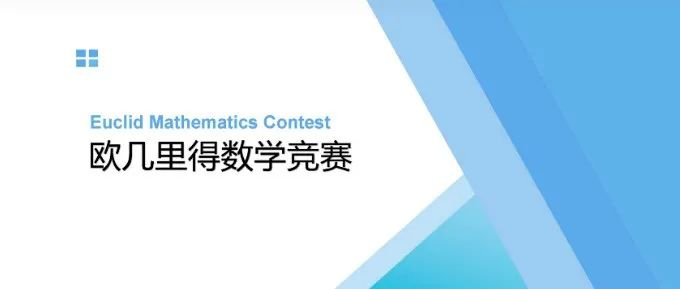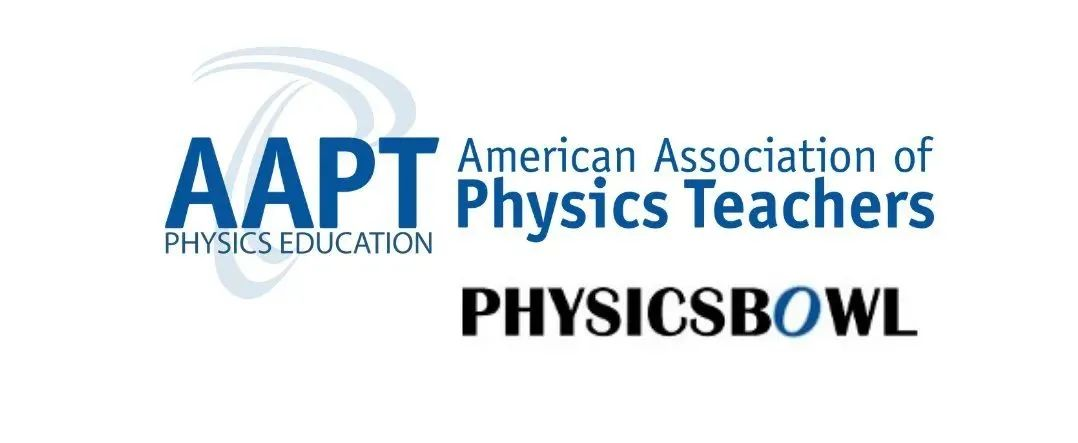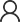# Euclid欧几里得竞赛一对一辅导课程

Euclid Contest（欧几里得竞赛）是加拿大滑铁卢大学（University of Waterloo）的数学院为全球高中生举办的数学竞赛，是加拿大最具认可度的数学竞赛。参加Euclid Contest对申请以数学和计算机著称的滑铁卢大学和该大学的奖学金很有帮助。University of Waterloo（滑铁卢大学）拥有加拿大唯一，也是北美最大的数学学院。滑铁卢大学的数学学院不仅有优质的教育，还有co-op（带薪实习）项目，所以吸引着来自世界各地的优秀学生。滑铁卢大学特别为全世界初中和高中生开设了Waterloo系列的数学竞赛，覆盖了7-12年级。

Gauss Contest：面向7－8年级

Pascal Contest，FryerContest ：面向9年级

Cayley Contest，Galois Contest ：面向10年级

Fermat Contest，Hypatia Contest ：面向11年级

Euclid Contest：面向12年级（低年级也可以参加）

Euclid欧几里得数学竞赛一对一辅导课程大纲

Number Theory

Main Topics

Prime factorization

Congruence and Modular Algebra

Digits and Base-n Representation

Diphantine Equations

Selected Essential Details (Materials with * are aimed for the potential last Problems)

Number of factors, Sum/Product of factors

LCM and GCD, *Euclidean Algorithm and Bézout's Theorem

Principles of Modular Calculations

*Euler’s Theorem/Fermat's Little Theorem

*Chinese Remainder Theorem(CRT)

Mutual Conversion between different bases

Estimation and Molular Method

Algebra

Main Topics

Sequences

Functions and Equations

Inequalities and Extreme Value Problems

Polynomials

Selected Essential Details (Materials with * are aimed for the potential last Problems)

Arithemetic and Geometric Sequences

Periodic Sequences, *Recursive Sequences and Characteristic Equation Method

*Conjecture and Mathematical Induction Proof

Elementary Functions (Linear, Quadratic, Exponential, Logarithmic, Trigonometric) and their properties

Functional Equations

*Gaussian/Floor function

Simple Polynomial Inequalities

AM-GM Inequality, *Cauchy inequality

Division Algorithm of Polynomials and the Remainder's Theorem

Fundamental Theorem of Algebra (Polynomial Factorization) and Vieta's Theorem

The Rational Root Theorem

Geometry

Main Topics

Triangles and Polygons

Circles

Basic Coordinate Geometry

Basic Solid Geometry

Selected Essential Details (Materials with * are aimed for the potential last Problems)

The Law of Sines, The Law of Cosines

Area Method and Heron's formula

*Menelaus's theorem, Ceva's theorem, Stewart Theorem

Centers of triangle

Chords, Arcs, Tangents, Inscribed and Central accepted angles

Power of a Point Theorem, *Ptolemy's theorem

Coordinate System and Equations of lines, Circles

Lines in space, Planes; Rectangular Box, Pyramids, Prisms, Sphere and Cones,Frustums

Combinatorics

Main Topics

Basic Counting Principle

Permutations and Combinations

Logic reasoning

Selected Essential Details (Materials with * are aimed for the potential last Problems)

Sum Rules and Product Rules

Combinatorics numbers and *Combinatorics identities

Grouping Theorems, Boards Method and the Problem of Balls into Boxes

*Pigeonhole principle### USABO/BBO生物竞赛备赛辅导课程5. ### IBDP课改后HL数学科目考什么？商务合作在线咨询